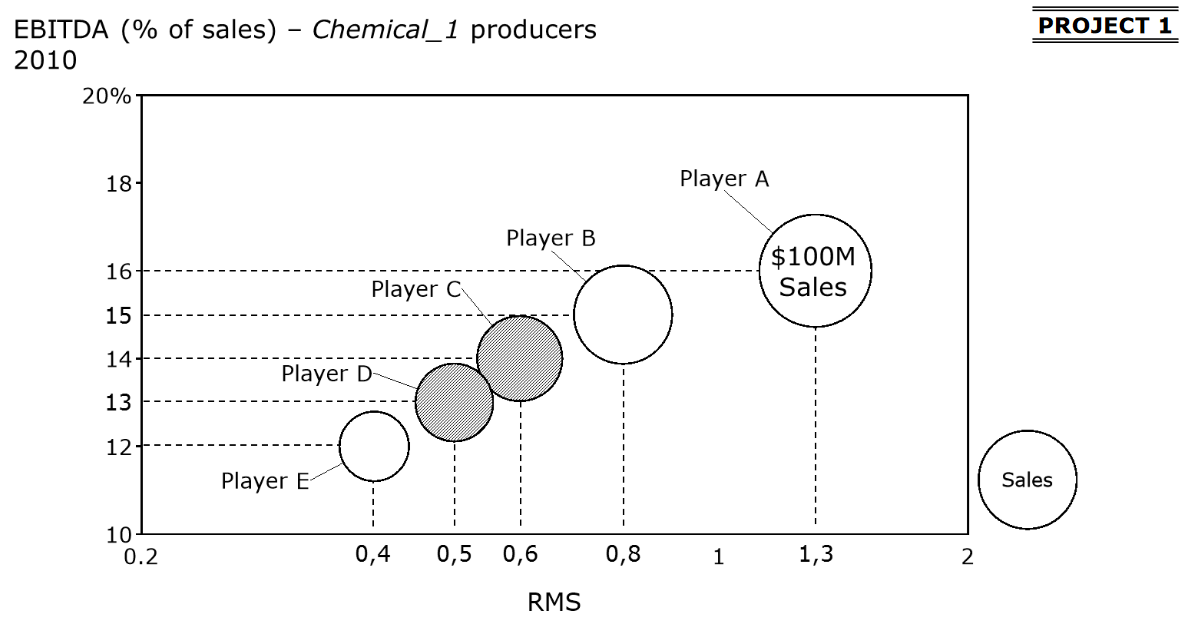# EBITDA% and RMS

business case case question
Letzte Aktivität am 24. März 2019
2 Antworten
2,0 Tsd. Aufrufe

I'm trying to figure out what would be, after the merge of Player C and D, the increase on the annual EBITDA, if they have the same EBITDA margin of Player A.

Based on the graph:
RMS Player A: 1.3
MS Player A: 1 / (0.4 + 0.5 + 0.6 + 0.8 + 1) = 0.3

MS Player C: 0.6 x 0.3 = 0.18
MS Player D: 0.5 x 0.3 = 0.15

So the merged MS will be 0.18 + 0.15 = 0.33 and is now the new market leader.

That means that the Player A RMS will be 0.3 / 0.33 = 0.9
Merged Player CD will have a new RMS 0.33 / 0.3 = 1.1

The problem that I have is that I don't know how to use the EBITDA % in these calculations.

How can I calculate the annual EBITDA using the EBITDA %?

That way I can calculate Player D with 0.4 RMS and 13%, Player C with 0.6 RMS and 14%, and Player CD with 1.1 RMS and 16%.

I know the answer for Player CD with 16% will be an increase of \$2.7M but how can I find that?
And does this increase of \$2.7M means that Player A lost \$2.7M with their new RMS?

Thanks in advance for any help!## Übersicht der Antworten

• Datum aufsteigend
• Datum absteigend
Beste Antwort

RMS Player A: 1.3

MS Player A: 1 / (0.4 + 0.5 + 0.6 + 0.8 + 1) = 0.3

MS Player C: 0.6 x 0.3 = 0.18

MS Player D: 0.5 x 0.3 = 0.15

Revenue C = (100M * 0.18) / 0.3 = 60M

Revenue D = (100M * 0.15) / 0.3 = 50M

Revenue CD = 110M

And using the formula for EBITDA

EBITDA% = EBITDA/Revenue

EBITDA C = 14% * 60 = 8.4

EBITDA D = 13% * 50 = 6.5

and calculating the EBITDA with the 16% from Player A

EBITDA CD = 16% * 110 = 17.6

The difference is 17.6 - 8.4 - 6.5 = \$2.7M

War diese Antwort hilfreich?

Hi, Just came across this question on PrepLounge. What formula did you use for Market Share? MS Player A: 1 / (0.4 + 0.5 + 0.6 + 0.8 + 1) = 0.3 (Isn't the formula to divide with company market share over the next biggest company market share? I don't understand where you got this formula from.

bump

Hi!

You made a good start.

Once you have the market shares for each player, you can use that to calculate their revenue, since you know the revenue for player a (100m). You don't need the new rms.

Now that you have the revenue you can use the ebitda margin to calculate the ebitda for the merged entity and the ebitda for player C and D (before the merger). The ebitda increase doesnt come from player A, but probably from efficiencies in the merged entity.

Hope this helps!

War diese Antwort hilfreich?